Examples for electric field and equipotential lines

Example #1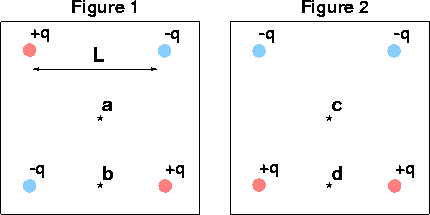Problem:

Is the electric field zero at a,b,c,d?

yes,no,no,no

Is the electric potential zero at a,b,c,d?

yes,yes,yes,no

Example #2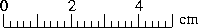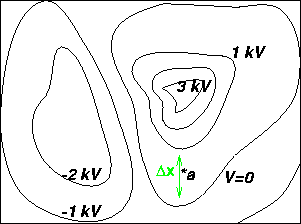Problem:

What is the magnitude and direction of the electric field at a?

Solution:

The electric field is given by: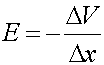where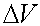is 1000 volts and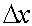is the distance between the two lines, which we measure to be 1.4 cm. Therefore E=1000/.014.

E = 7.1E4 (V)

Electric fields index